## Why do the coefficients of cross-sectional fixed effects and time fixed effects become zero?

I currently have a panel data set that contains the quarterly increment of loans initiated from more than 300 cities in China over the period from 2011Q1 to 2020Q2. I want to examine the impact of COVID-19 on lending activities. I believe that both time fixed effects and city fixed effects exist—both the F test for poolability and Hausman Test support fixed-effect model.
I use the panel procedure to analyze it. I also set a dummy variable, "event", to indicate whether the pandemic has occurred. The dummy variable "event" equals to 1 if the period is 2020Q1 or 2020Q2. Currently, I have not added any interactive terms.
If I specify a one-way model using fixone option that only allows cross-section fixed effects, I found that some coefficients of these fixed effects are significant while some are not. The coefficients of the dummy variable "event" and other control variables are also significant. By now, it is acceptable.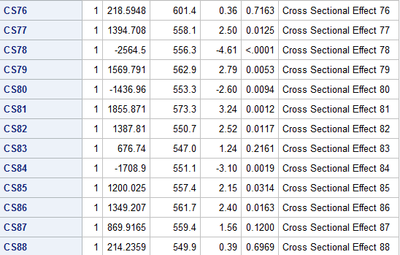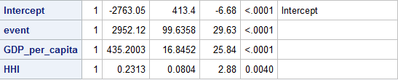However, if I specify a one-way model using fixonetime option that only allows time fixed effects, it will be a mess. Although the coefficients of all time fixed effects are significant, the coefficient of the dummy variable "event" becomes zero. I am wondering whether it is caused by perfect multicollinearity. After all, in my setting, event = 1 means the same thing as the fixed effects of 2020Q1 and 2020Q2. My guess is that the impact of the dummy variable "event" has already been absorbed by time fixed effects. Is that right? If it is correct, does it mean that you can never use the time fixed effects and some dummy variables indicating certain event happens at the same time? However, several papers are studying the impact of COVID-19 using them simultaneously in their model. They did not mention any problems of such a setting. So I feel confused.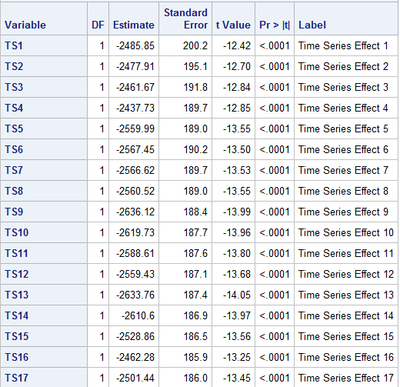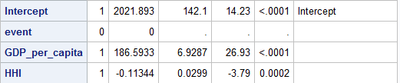In a branched setting, instead of using the dummy variable "event", I use two dummy variable, "event_level1" and "event_level2", which equals to 1 if the period is 2020Q1 and 2020Q2 respectively, to visualize the dynamic of COVID-19 impact. The result is getting worse because the coefficients of all fixed effects and interested dummy variables "event_level1" and "event_level2" become zero this time. It looks caused by other reasons instead of multicollinearity this time. Are there any generous and smart friends who know the reason? Thank you so much!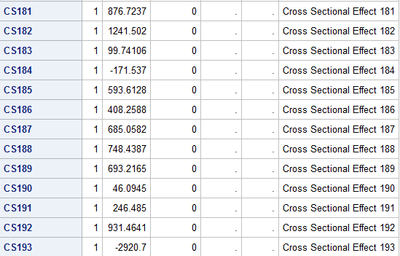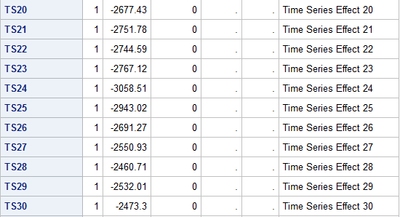9 REPLIES 9SteveDenham

## Re: Why do the coefficients of cross-sectional fixed effects and time fixed effects become zero?

You called out the problem yourself.  The event variable (and later the event1 and event2 variables) is perfectly associated with your time variable, and the results are what you see.  The way to address this is to use the TEST statement to construct comparisons of interest between the coefficients of the quarter variables, and not include 'event' in the MODEL statement.

SteveDenham

## Re: Why do the coefficients of cross-sectional fixed effects and time fixed effects become zero?

Thank you very much for your suggestions. I will study the test statement. But by now, based on what I have know, I guess your suggestion means that I cannot include both time fixed effects and other dummy variables indicating whether interested event happens in the same model. Am I understanding it correctly?SteveDenham

## Re: Why do the coefficients of cross-sectional fixed effects and time fixed effects become zero?

That is correct.  However, since your other variables can be expressed as linear functions of the time fixed effects (exact collinearity), the TEST statement provides a way to get a Wald chi squared test.  From the chisquared value and the known mean difference, you could get an approximation of the standard deviation of the difference.  You should automatically get a probability.

For instance, let's suppose that your time series covers just 4 quarters, and an intervention occurs such that the last two quarters are affected.  Then the following provides a test of the existence of an effect:

```MODEL result = q1 q2 q3 q4;
TEST (q1 + q2) - (q3 + q4) = 0;```

If you can share your MODEL statement, I am sure that an appropriate TEST statement can be devised.

SteveDenham

## Re: Why do the coefficients of cross-sectional fixed effects and time fixed effects become zero?

The code to get the first two pictures is:

```/*
R square = 0.2854
F test for no fixed effects p < 0.0001
Some cross-section fixed effects are significant, some are not
beta_event_level1 = 3855.073 p < 0.0001
beta_event_level2 = 2038.478 p < 0.0001
beta_GDP_per_capita = 434.9171 p <0.0001
beta_HHI = 0.231173 p = 0.0039
*/
proc panel data=rrd_come.city_ln_combo_8;
id City TimeID;
model LoanNum = event_level1 event_level2 GDP_per_capita HHI / fixone printfixed;
run;```

The code to get the middle two pictures is:

```/*
R square = 0.2886
F test for no fixed effects p < 0.0001
All time fixed effects are significant
beta_event = 0
beta_GDP_per_capita = 186.5933 p <0.0001
beta_HHI = -0.11344 p = 0.0002
*/
proc panel data=rrd_come.city_ln_combo_8;
id City TimeID;
model LoanNum = event GDP_per_capita HHI / fixonetime printfixed;
run;```

The code to get the last three pictures is:

```/*
R square = 0.4044
F test for no fixed effects p < 0.0001
All cross-section and time fixed effects are zero and insignificant
beta_event_level1 = -4.39E12 p = 0.9997
beta_event_level2 = 0
beta_GDP_per_capita = 329.8557 p < 0.0001
beta_HHI = 0.505056 p <0.0001
*/
proc panel data=rrd_come.city_ln_combo_8;
id City TimeID;
model LoanNum = event_level1 event_level2 GDP_per_capita HHI / fixtwo printfixed;
run;```

## Re: Why do the coefficients of cross-sectional fixed effects and time fixed effects become zero?

Code?

Code is important to tell us the procedure, so we have a chance of knowing what bits are used, and the options you specify almost certainly have an impact.

Best to post the code in a code box opened on the forum with either the </> or "running man" icon.

## Re: Why do the coefficients of cross-sectional fixed effects and time fixed effects become zero?

The code to get the first two pictures is:

```/*
R square = 0.2854
F test for no fixed effects p < 0.0001
Some cross-section fixed effects are significant, some are not
beta_event_level1 = 3855.073 p < 0.0001
beta_event_level2 = 2038.478 p < 0.0001
beta_GDP_per_capita = 434.9171 p <0.0001
beta_HHI = 0.231173 p = 0.0039
*/
proc panel data=rrd_come.city_ln_combo_8;
id City TimeID;
model LoanNum = event_level1 event_level2 GDP_per_capita HHI / fixone printfixed;
run;```

The code to get the middle two pictures is:

```/*
R square = 0.2886
F test for no fixed effects p < 0.0001
All time fixed effects are significant
beta_event = 0
beta_GDP_per_capita = 186.5933 p <0.0001
beta_HHI = -0.11344 p = 0.0002
*/
proc panel data=rrd_come.city_ln_combo_8;
id City TimeID;
model LoanNum = event GDP_per_capita HHI / fixonetime printfixed;
run;```

The code to get the last three pictures is:

```/*
R square = 0.4044
F test for no fixed effects p < 0.0001
All cross-section and time fixed effects are zero and insignificant
beta_event_level1 = -4.39E12 p = 0.9997
beta_event_level2 = 0
beta_GDP_per_capita = 329.8557 p < 0.0001
beta_HHI = 0.505056 p <0.0001
*/
proc panel data=rrd_come.city_ln_combo_8;
id City TimeID;
model LoanNum = event_level1 event_level2 GDP_per_capita HHI / fixtwo printfixed;
run;  ```SteveDenham

## Re: Why do the coefficients of cross-sectional fixed effects and time fixed effects become zero?

Type 3 error on my part of answering the wrong question. It is obvious that event is confounded with TimeID - TimeID is the basis of the definition of the event variable.  So, you can't have a regressor in the model that is a function of either the panel-id or the timeseries-id.  To see this create a 2 level variable from City (say Region). Include that variable in your fixone model.  It ought to come back with 0 df.

.So what alternatives are there?  Well, you could fit a repeated measures model using PROC MIXED or GLIMMIX.  That would eliminate many of the specific tests available in PROC PANEL, but would enable you to estimate the event size, standard error and p value.

``````proc glimmixl data=rrd_come.city_ln_combo_8;
class City TimeID;
model LoanNum =TimeID GDP_per_capita HHI / dist=poisson;
lsmestimate TimeID 'event' -0.5 -0.5 0.5 0.5/ilink cl;
run;``````

There are some assumptions here.  First is that TimeID has 4 levels, and 'event' occurs during the latter two and not in the first two.  Second, that LoanNum is a count of the number of loans per city per TimeID.  Third, that the variance over time is homogeneous.  Fourth, that the time effect is fixed, such that its influence on.the model can be attributed to correlation between the residuals in addition to the estimated values at each TimeID.

The least squares means are calculated at the mean value of GDP_per_capita and HHI.  If you want estimates at other specified values, you will need additional LSMEANS statements with the AT option. Similar adjustments would be made for the LSMESTIMATE statement.

SteveDenham

## Re: Why do the coefficients of cross-sectional fixed effects and time fixed effects become zero?

I cannot quite understand the advantages of proc panle and proc glmmix over proc panel. In other words, what is the major problem if I use proc panel to analyze my case?SteveDenham

## Re: Why do the coefficients of cross-sectional fixed effects and time fixed effects become zero?

Why do I suggest GLIMMIX over PANEL?  Mostly so you can get a test for elements that are completely confounded with time series in PANEL.  In PANEL, I don't see a way to test for level shifts or time trends - it assumes stationarity in some form or another.

SteveDenham

Discussion stats
• 9 replies
• 591 views
• 0 likes
• 3 in conversation